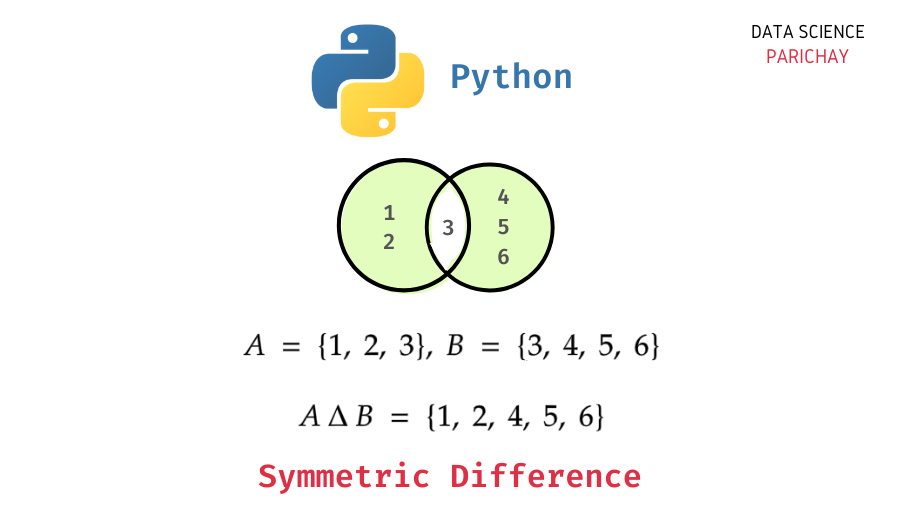# Set Symmetric Difference in Python

In this tutorial, we will look at how to calculate the symmetric difference between two sets in Python with the help of some examples.

The symmetric difference between two sets (for example, A and B) is the set of values from both sets that are not shared between the two sets. That is values that are in either set A or set B but not in both. Let’s look at an example.

You can see that the resulting set contains values that are present in either A or B but not in both sets.

Python comes with a built-in set data structure to implement a set. It also has a number of additional functions to help you with common operations on sets such as union, intersection, difference, etc.

To get the symmetric difference between two sets in Python, you can use the set `symmetric_ difference()` function. The following is the syntax:

```# symmertic difference between a and b
a.symmetric_difference(b)```

It returns a new set containing the elements unique to either a or b and not including the elements shared by the two sets.

```# create two sets
a = {1,2,3}
b = {2,3,4,5}
# symmetric difference
a.symmetric_difference(b)```

Output:

`{1, 4, 5}`

We get the resulting set as {1, 4, 5} as these are the elements that are not shared between the two sets. Elements 2 and 3 are common between a and b.

📚 Data Science Programs By Skill Level

Introductory

Intermediate ⭐⭐⭐

🔎 Find Data Science Programs 👨‍💻 111,889 already enrolled

Disclaimer: Data Science Parichay is reader supported. When you purchase a course through a link on this site, we may earn a small commission at no additional cost to you. Earned commissions help support this website and its team of writers.

Alternatively, you can also use the `^` operator to compute symmetric differences between sets in Python. For example,

```# create two sets
a = {1,2,3}
b = {2,3,4,5}
# symmetric difference
a ^ b```

Output:

`{1, 4, 5}`

We get the same result as above.

Note that the `^` operator for the symmetric difference only works on sets whereas you can pass other iterables like lists, tuples, etc. to the set `symmertic_difference()` function.

You might also be interested in –

•